# Thinness of a set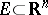at a pointA local criterion for the fact thatis a polar set. A non-empty setis said to be thin at the pointin two cases:

1)is not a limit point of; that is,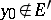, whereis the derived set of;

2)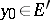and there exists a superharmonic function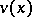in a neighbourhood of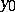(see Superharmonic function) such that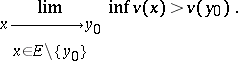The setis polar if and only if it is thin at each of its points. For an arbitrary setthe subset of those points at whichis thin is polar. Any non-empty subset of a set which is thin at the point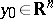is thin at. The union of a finite number of sets thin at the pointis a set thin at.

A segment in the planeis not a thin set at any of its points. If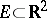is a thin set at a point, then there exist arbitrarily small discs with centreand not intersecting. A polar setis completely discontinuous. However, the Cantor set on the-axis (which is of measure zero) is not thin at any of its points. At the same time, for example, inthe set of pointswhich has a spine at the point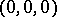, where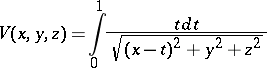is the Newton potential with densityon the segment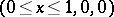, is a thin set at the spine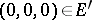(Lebesgue's example).